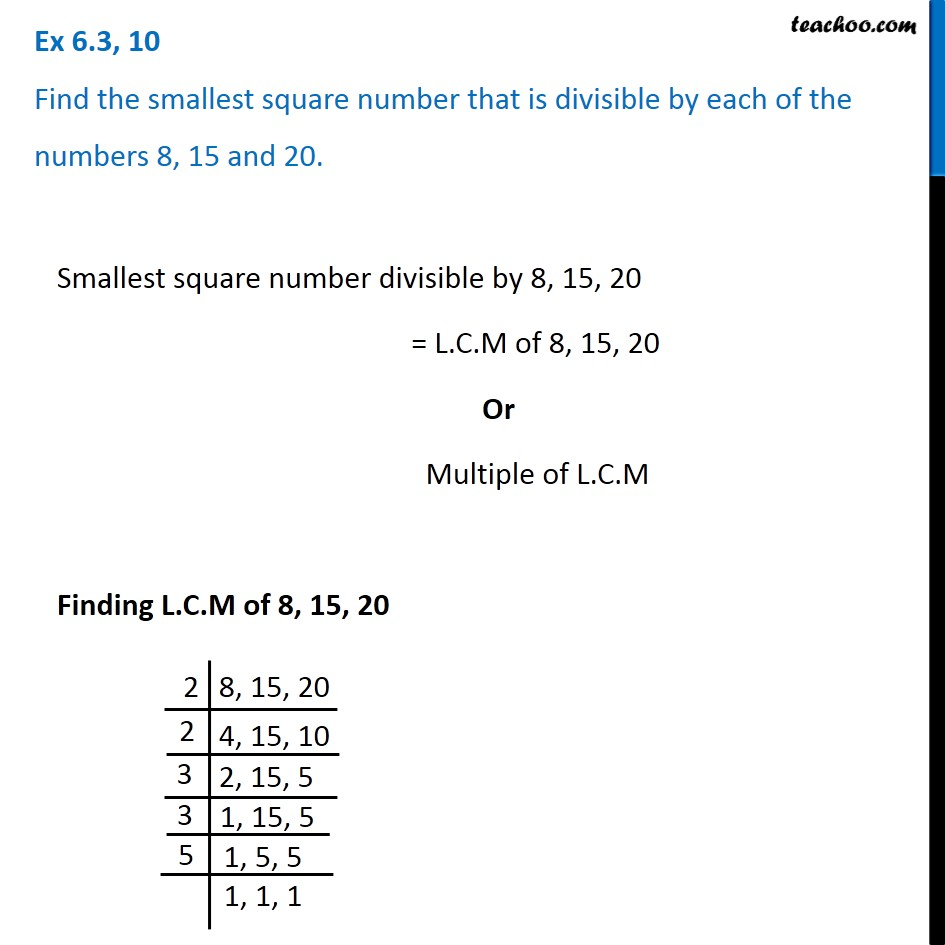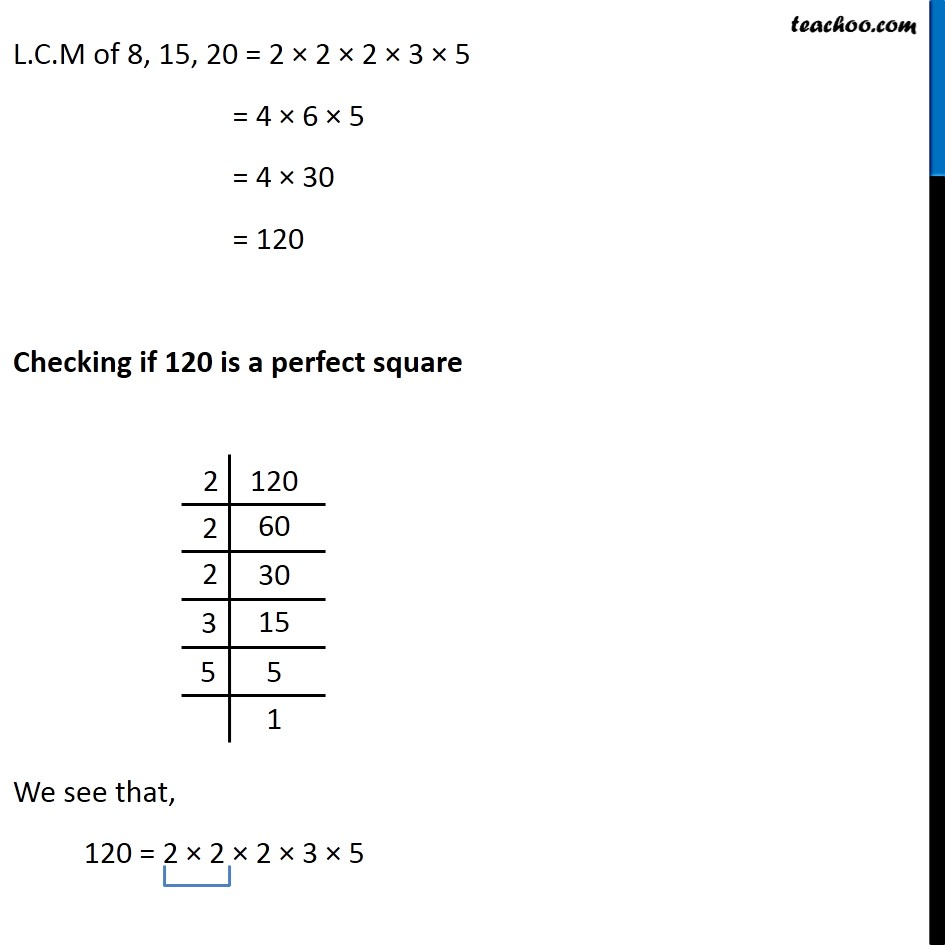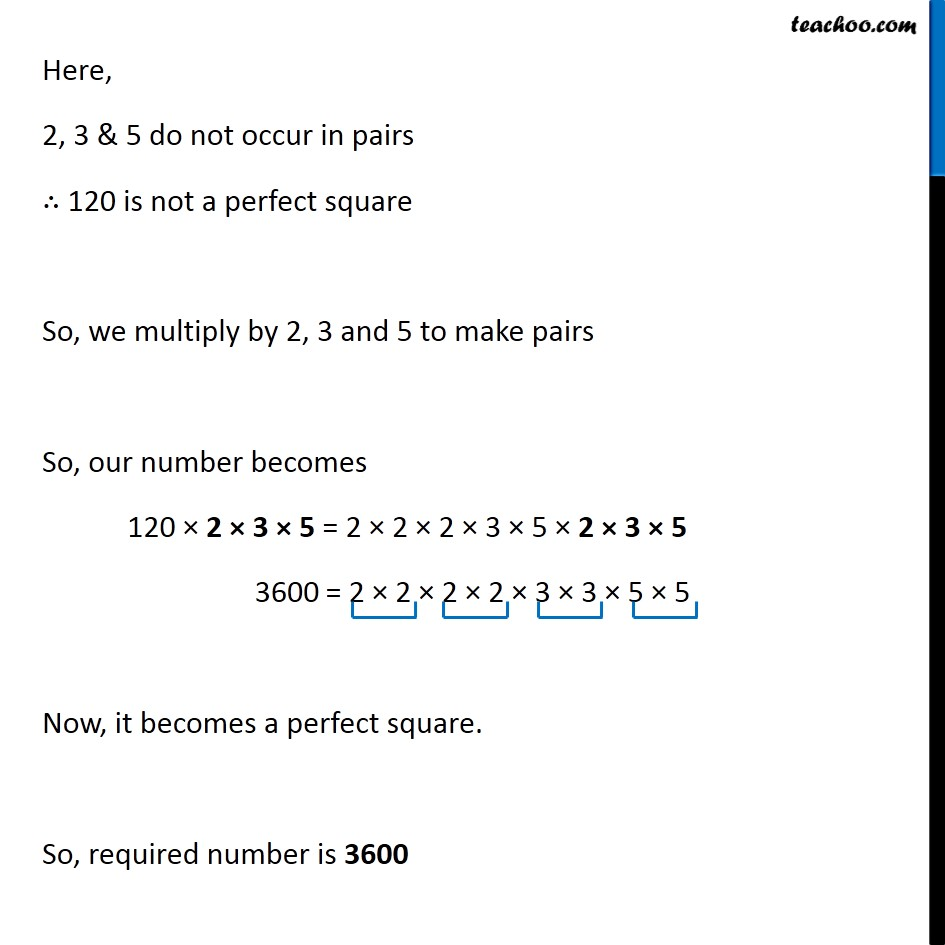Smallest square number divisible by numbers

Chapter 6 Class 8 Squares and Square Roots
Concept wiseIntroducing your new favourite teacher - Teachoo Black, at only ₹83 per month

### Transcript

Ex 6.3, 10 Find the smallest square number that is divisible by each of the numbers 8, 15 and 20.Smallest square number divisible by 8, 15, 20 = L.C.M of 8, 15, 20 Or Multiple of L.C.M Finding L.C.M of 8, 15, 20 L.C.M of 8, 15, 20 = 2 × 2 × 2 × 3 × 5 = 4 × 6 × 5 = 4 × 30 = 120 Checking if 120 is a perfect square We see that, 120 = 2 × 2 × 2 × 3 × 5 Here, 2, 3 & 5 do not occur in pairs ∴ 120 is not a perfect square So, we multiply by 2, 3 and 5 to make pairs So, our number becomes 120 × 2 × 3 × 5 = 2 × 2 × 2 × 3 × 5 × 2 × 3 × 5 3600 = 2 × 2 × 2 × 2 × 3 × 3 × 5 × 5 Now, it becomes a perfect square. So, required number is 3600